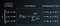Activation Functions

A neural network is a network of artificial neurons connected to each other in a specific way. Job of neural network is to learn from given data. The prediction function that neural network must learn can be highly non-linear. Activation functions for artificial neurons are chosen to capture underlying non-linearity.

Activation functions (generally) have functional form of f(u)=f(w ᵀ x+b), where w is weight vector and x single training data vector

This can be treated as linear combination of inputs, followed by a non-linear transformation. There are multitude of options available to chose a non-linear transformation. Some of the prominent ones are as follows.

1. Sigmoid Activation Function

A sigmoid function, f(u)= 1 / (1 + e⁻ ᵘ). It takes a real-valued number and “squeeze” it into range between 0 and 1. Large negative numbers become ≈0 and large positive numbers become ≈1.

Pros:

For binary classification problem it is used as activation of output layer of a neural network.

Cons:

1. Can saturate and kill gradients: When neuron’s activation saturates at 1 or 0 , the gradient becomes almost zero. This creates difficulties in learning.
2. Outputs are not zero-centered: Since outputs are in range 0 to 1, neurons in next layer will receive data that is not zero centered. Hence, gradient of weights w during backpropagation will be either all positive or all negative, which can cause undesirable zig-zagging dynamics in gradient updates of weights. When considering gradients added over all training data in a batch, this problem will not be much severe compared to “Saturate and kill gradients”

2. Tanh Activation Function

A tanh function, f(u)=sinh(u)/cosh(u). It takes a real-valued number and “squeeze” it into range between -1 and 1. Large negative numbers become ≈−1 and large positive numbers become≈1.

Pros:

It is preferred over sigmoid because its outputs are zero centered

Cons:

Can saturate and kill gradients: When neuron’s activation saturates at 1 or -1 , the gradient becomes almost zero. This creates difficulties in learning.

3. ReLU Activation Function

The Rectified Linear Unit, ReLU is f(u)=max(0,u)

Pros:

1. Greatly increase training speed compared to tanh and sigmoid
2. Less expensive computations compared to tanh and sigmoid
3. Reduces likelihood of the gradient to vanish. Since when u>0, the gradient has constant value.
4. Sparsity: When more u<=0, the f(u) can be more sparse

Cons:

1. Tends to blow up activation (there is no mechanism to constrain the output of the neuron, as u itself is the output).
2. Closed ReLU or Dead ReLU: If inputs tend to make u<=0, then the most of the neurons will always have 0 gradient updates hence closed or dead.

4. Leaky ReLU:

It solves the dead ReLU problem. 0.01 is coefficient of leakage. Leaky ReLU is as follows:

5. Parameterized ReLU Or PReLU:

Parameterizes coefficient of leakage αα in Leaky ReLU.

6. Maxout

Generalization of ReLU, Leaky ReLU and PReLU. It does not have functional form of f(u)=f(w ᵀ x+b) , instead it computes function max(w′ᵀx+b′,wᵀx+b)

Pros:

Maxout has pros of ReLU but doesn’t have dead ReLU issue

Cons:

It has twice number of weight parameters to learn w′ and w

7. SoftmaxUdacity Deep Learning Slide on Softmax

A sofmax function is generalization of sigmoid function. Sigmoid is used for 2-class (binary) classification whereas Softmax is used for multi-class classification. As shown in above figure Softmax function turns logits [2.0, 1.0, 0.1] into probabilities [0.7, 0.2, 0.1]

What Activation Function Should I Use ?

1. For output layer, use sigmoid or softmax in classification task
2. For output layer, use no activation or Purelin function f(u)=u in regression task
3. Use the ReLU non-linearity, if you carefully set learning rates and monitor the fraction of “dead ReLU” in network.
4. Else try Leaky ReLU or Maxout.
5. Or try tanh, although it might be worse than ReLU
6. Avoid sigmoid

Differentiation:

Basic Formulas:

Given f(x)f(x) and g(x)g(x) are differentiable functions (the derivative exists), cc and nn are any real numbers:

References:

1. Tensorflow playground link
2. http://cs231n.github.io
3. Udacity Deep Learning Slide on Softmax

Written by

Rakesh Malviya

Using technology, data and design to change the way the world shops. Learn more about us - http://walmartlabs.com/

Welcome to a place where words matter. On Medium, smart voices and original ideas take center stage - with no ads in sight. Watch
Follow all the topics you care about, and we’ll deliver the best stories for you to your homepage and inbox. Explore
Get unlimited access to the best stories on Medium — and support writers while you’re at it. Just \$5/month. Upgrade